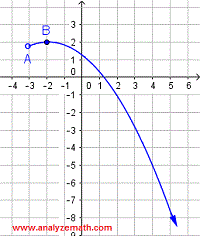# Solutions to Questions on Domain and Range of Relations

Detailed solutions and explanations to questions on how find the domain and range of a relation are presented.

## Questions and Solutions

Find the domain and range for each relation given by its graph, and state whether the relation is a function.

### Question 1### Solution

a)
Domain: Points A(-8 , - 0.5) and B(4,0) have the smallest and the largest x-coordinate respectively. The domain is the set of all x values between the smallest x-coordinate (that of A) to the largest x-coordinate (that of B) and is written as:
- 8 ≤ x ≤ 4
Since the relation is defined at both points (closed circle) the inequality symbol ≤ is used.
b)
Range: Points C(-3,-5) and B(4,0) have the smallest and largest y-coordinates respectively. Hence, the range is the set of all y values between the smallest and the largest y coordinates and is given by the double inequality:
- 5 ≤ y ≤ 0
The inequality symbol ≤ is used at both sides the relation is defined at these y values (closed circles).
c)
The relation graphed above is a function because no vertical line can intersect the given graph at more than one point.

### Question 2### Solution

a)
Domain: Points A(-2 , 4) and B(4,6) have the smallest and the largest x-coordinate respectively. The domain is the set of all x values from the smallest x-coordinate (that of A) to the largest x-coordinate (that of B) and is written as:
- 2 ≤ x ≤ 4
Closed circles at both points A and B hence the use of the inequality symbol ≤.
b)
Range: Points C(2,-2) and B(4,6) have the smallest and largest y-coordinates respectively. Hence, the range is the set of all y values between the smallest and the largest y coordinates and is given by the double inequality:
- 2 ≤ y ≤ 6
The inequality symbol ≤ is used at both sides the relation is defined at these y values (closed circle).
c)
The relation graphed above is a function because no vertical line can intersect the given graph at more than one point.

### Question 3### Solution

a)
Domain: Point A(4 , 2) has the largest x-coordinate. As x decreases (moving left), the arrow at the top left indicates that there is no limit to the smallest value of the x-coordinate of any point on the given graph. The domain is the set of all x values smaller than 4 and is written as:
x ≤ 4
The closed circle at point A means the relation is defined at x = 4, hence the use of the inequality symbol ≤.
b)
Range: Points B(2,-2) and C(-2,-2) have the smallest (and equal) y-coordinates. The arrow on the top left indicates that as x decreases (moving left), the y coordinate of points on the graph increases without limit. Hence, the range is the set of all y values greater than or equal to -2 and is given by the inequality:
y ≥ -2
The inequality symbol ≤ is used because the relation is defined at y = 4 (closed circle).
c)
The relation graphed above is a function because no vertical line can intersect the given graph at more than one point.

### Question 4### Solution

a)
Domain: Points A(-5 , -1) and B(1, -1) have the smallest and the largest x-coordinate respectively. The domain is the set of all x values between - 5 and - 1 and is given by:
- 5 ≤ x ≤ 1
The closed circle at points A and B means the relation is defined at x = - 5 and x = 1, hence the use of the inequality symbol ≤ at both sides.
b)
Range: Points C(-2,-3) and D(-2,1) have the smallest and the largest y-coordinates respectively. Hence, the range is the set of all y values between -3 and 1 and is given by:
-3 ≤ y ≤ 1
The inequality symbol ≤ is used because the relation is defined at both points (closed circle).
c)
The relation graphed above is NOT a function because at least one vertical line intersects the given graph at two points as shown below.### Question 5### Solution

a)
Domain: Point A(-3 , 1.8) has the smallest x-coordinate. As x increases (moving right), the arrow at the bottom right, indicates that there is no limit to the largest value of the x-coordinate of any point on the given graph. The domain is the set of all x values greater than -3 and is written as:
x > -3
The open circle at point A means the relation is not defined at x = -3, hence the use of the inequality symbol >.
b)
Range: Points B(-2,2) have the largest y-coordinates. The arrow on the bottom right indicates that as x increases (moving right), the y coordinate of points on the graph decreases without limit. Hence, the range is the set of all y values smaller than or equal to 2 and is given by the inequality:
y ≤ 2
The inequality symbol ≤ is used because the relation is defined at y = 2 (closed circle at B).
c)
The relation graphed above is a function because no vertical line can intersect the given graph at more than one point.